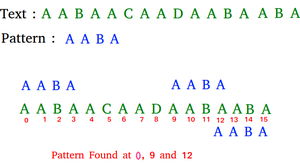# Python Program for Rabin-Karp Algorithm for Pattern Searching

Given a text txt[0..n-1] and a pattern pat[0..m-1], write a function search(char pat[], char txt[]) that prints all occurrences of pat[] in txt[]. You may assume that n > m.
Examples:

```Input:  txt[] = "THIS IS A TEST TEXT"
pat[] = "TEST"
Output: Pattern found at index 10

pat[] =  "AABA"
Output: Pattern found at index 0
Pattern found at index 9
Pattern found at index 12```` `

The Naive String Matching algorithm slides the pattern one by one. After each slide, one by one checks characters at the current shift, and if all characters match then print the match. Like the Naive Algorithm, the Rabin-Karp algorithm also slides the pattern one by one. But unlike the Naive algorithm, the Rabin Karp algorithm matches the hash value of the pattern with the hash value of the current substring of text, and if the hash values match then only it starts matching individual characters. So Rabin Karp’s algorithm needs to calculate hash values for the following strings.1) Pattern itself.2) All the substrings of the text of length m.

## Python

 `# Following program is the python implementation of` `# Rabin Karp Algorithm given in CLRS book`   `# d is the number of characters in the input alphabet` `d ``=` `256`   `# pat  -> pattern` `# txt  -> text` `# q    -> A prime number`   `def` `search(pat, txt, q):` `    ``M ``=` `len``(pat)` `    ``N ``=` `len``(txt)` `    ``i ``=` `0` `    ``j ``=` `0` `    ``p ``=` `0`    `# hash value for pattern` `    ``t ``=` `0`    `# hash value for txt` `    ``h ``=` `1`   `    ``# The value of h would be "pow(d, M-1)% q"` `    ``for` `i ``in` `xrange``(M``-``1``):` `        ``h ``=` `(h ``*` `d)``%` `q`   `    ``# Calculate the hash value of pattern and first window` `    ``# of text` `    ``for` `i ``in` `xrange``(M):` `        ``p ``=` `(d ``*` `p ``+` `ord``(pat[i]))``%` `q` `        ``t ``=` `(d ``*` `t ``+` `ord``(txt[i]))``%` `q`   `    ``# Slide the pattern over text one by one` `    ``for` `i ``in` `xrange``(N``-``M ``+` `1``):` `        ``# Check the hash values of current window of text and` `        ``# pattern if the hash values match then only check` `        ``# for characters one by one` `        ``if` `p ``=``=` `t:` `            ``# Check for characters one by one` `            ``for` `j ``in` `xrange``(M):` `                ``if` `txt[i ``+` `j] !``=` `pat[j]:` `                    ``break`   `            ``j``+``=` `1` `            ``# if p == t and pat[0...M-1] = txt[i, i + 1, ...i + M-1]` `            ``if` `j ``=``=` `M:` `                ``print` `"Pattern found at index "` `+` `str``(i)`   `        ``# Calculate hash value for next window of text: Remove` `        ``# leading digit, add trailing digit` `        ``if` `i < N``-``M:` `            ``t ``=` `(d``*``(t``-``ord``(txt[i])``*``h) ``+` `ord``(txt[i ``+` `M]))``%` `q`   `            ``# We might get negative values of t, converting it to` `            ``# positive` `            ``if` `t < ``0``:` `                ``t ``=` `t ``+` `q`   `# Driver program to test the above function` `txt ``=` `"GEEKS FOR GEEKS"` `pat ``=` `"GEEK"` `q ``=` `101` `# A prime number` `search(pat, txt, q)`   `# This code is contributed by Bhavya Jain`

Output:

```Pattern found at index 0
Pattern found at index 10```

Time Complexity: O(nm), where m is the length of the pattern and n is the length
Auxiliary Space: O(1).

Please refer complete article on Rabin-Karp Algorithm for Pattern Searching for more details!

Whether you're preparing for your first job interview or aiming to upskill in this ever-evolving tech landscape, GeeksforGeeks Courses are your key to success. We provide top-quality content at affordable prices, all geared towards accelerating your growth in a time-bound manner. Join the millions we've already empowered, and we're here to do the same for you. Don't miss out - check it out now!